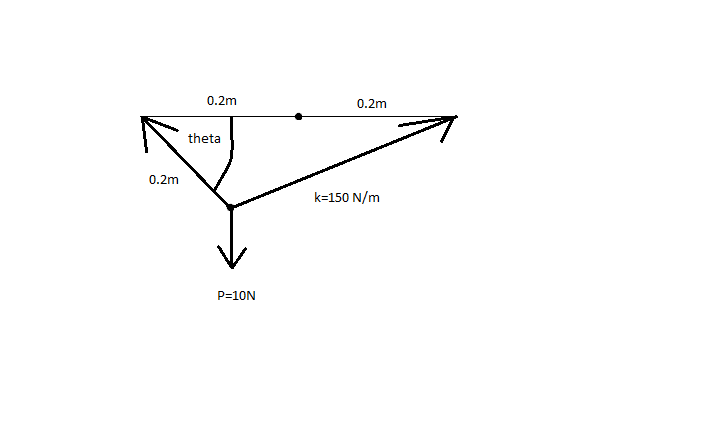User Name Remember Me? Password

 Equilibrium and Elasticity Equilibrium and Elasticity Physics Help ForumOct 13th 2010, 09:55 PM #1 Junior Member   Join Date: Sep 2010 Posts: 24 Difficult equilibrium problem 1. The problem statement, all variables and given/known data A vertical force P=10 N is applied to the ends of the 0.2 m cord AB and spring AC. If the spring has an unstretched length of 0.2 m. determine the angle theta for equilibrium. Take k = 150 N/m. [IMG]http://i49.photobucket.com/albums/f272/dranseth/****.png?t=1287031089[/IMG] 2. Relevant equations Fspring=ks Fy=0 Fx=0 3. The attempt at a solution Any of my attempts at this solution have resulted in pages and pages of work. I really am not seeing a short cut as to find the angle theta. Thanks for your help!Oct 14th 2010, 08:36 AM #2 Physics Team   Join Date: Jun 2010 Location: Mauritius Posts: 609 Your image is not appearing and the link leads to nowhere near the image... Could you try to have another link (look for "Link for message boards")? __________________ Jerry (Got my results!) It is easier to protect your feet with slippers than to cover the earth with carpet. No one can go back and change a bad beginning; but anyone can start now and create a successful ending. If a problem can be solved, no need to worry about it. If it cannot be solved what is the use of worrying?Oct 15th 2010, 02:44 PM   #3
Junior Member

Join Date: Sep 2010
Posts: 24
 Originally Posted by Unknown008Your image is not appearing and the link leads to nowhere near the image... Could you try to have another link (look for "Link for message boards")?Oct 16th 2010, 12:50 AM #4 Physics Team   Join Date: Jun 2010 Location: Mauritius Posts: 609 Hm... let me clarify, that's it?I can't find any shortcut.The length of the spring is given by l + e, where e = F/k, e = F/150 So, length of spring = (0.2 + F/150) m Let Tc be the tension in the cord and Ts be the tension in the spring. (I'll use B as the angle instead) Tc sinB + Ts sinC = 10 where C is the angle BCA. Tc cosB = Ts cosC From the cosine rule, we get: AC^2 = BC^2 + AB^2 - 2(AB)(BC)cos B (0.2 + F/150)^2 = 0.4^2 + 0.2^2 - 2(0.4)(0.2)cos B (0.2 + F/150)^2 = 0.2 - 0.16cos B Now, F = Ts Using the first two equations, we get: ((Ts cosC)/cosB)sinB + Ts sinC = 10 Ts cosC tanB + Ts sinC = 10 Ts = 10/(cosC tanB + sinC) Hence, [0.2 + 10/{150(cosC tanB + sinC)}]^2 = 0.2 - 0.16cos B Hm... not very 'pretty'. Let's try the sine rule. [0.2 + 10/{150(cosC tanB + sinC)}/sinB = 0.2/sinC = 0.4/sinA [0.2 + 10/{150(cosC tanB + sinC)} = (0.2 sinB)/sinC So, 0.2 - 0.16cos B = ((0.2 sinB)/sinC)^2 0.2 - 0.16cos B = (0.04 sin^2B)/sin^2C 5 - 4cos B = sin^2B/sin^2C We have: 5 - 4cos B = sin^2B/sin^2C 0.2/sinC = 0.4/sinA A + B + C = 180 Hmm....Maybe trial and error?__________________ Jerry (Got my results!) It is easier to protect your feet with slippers than to cover the earth with carpet. No one can go back and change a bad beginning; but anyone can start now and create a successful ending. If a problem can be solved, no need to worry about it. If it cannot be solved what is the use of worrying?Tags difficult, equilibrium, problemThread ToolsShow Printable VersionEmail this Page Display ModesLinear ModeSwitch to Hybrid ModeSwitch to Threaded ModeSimilar Physics Forum Discussions Thread Thread Starter Forum Replies Last Post xtheunknown0 Equilibrium and Elasticity 6 Jun 13th 2013 03:57 AM LittleWing Kinematics and Dynamics 9 Feb 23rd 2011 08:19 AM Zachary Equilibrium and Elasticity 1 Aug 6th 2009 01:55 AM werehk Kinematics and Dynamics 2 Sep 9th 2008 08:44 AM baz00ka760 Equilibrium and Elasticity 1 Apr 24th 2008 01:04 PM